Function Repository Resource:

# DirectionalDerivativePlot3D

Visualize the directional derivative in a 3D plot

Contributed by: Dennis M Schneider
 ResourceFunction["DirectionalDerivativePlot3D"][f,{x,xmin,xmax},{y,ymin,ymax},pt,v]]] returns a plot of f along with a plot of the directional derivative vector at the point pt in the direction of the vector v. Several points and/or vectors can be given in a list.

## Details and Options

Setting "SectionStyle"Tube will suppress drawing the section.
Setting "DrawGraph"False will suppress the plot and return the directional derivative.
ResourceFunction["DirectionalDerivativePlot3D"] has the following options in addition to those for the resource function SectionPlot3D and Plot3D:
 "ArrowSize" Medium size of the arrowhead "BaseVectorPosition" Automatic determines the position of the base vector if drawn "DrawBaseVector" False whether to draw the base vector "DrawGraph" True whether to include the plot of the surface "DrawPlane" False whether to include the plane determining the section "PointStyle" PointSize[Large] graphics directive to specify the style for the point(s) "PrintDisplay" False whether to print the value of the directional derivative "SectionStyle" {{Tube[0.01],Black}} style for the section determined by the direction vector "UseLimit" False whether to use the limit definition to calculate the directional derivative "VectorStyle" {{Thickness[Medium],Red}} graphics directive to specify the style for the vector(s)

## Examples

### Basic Examples (1)

Visualize a directional derivative:

 In:=Out=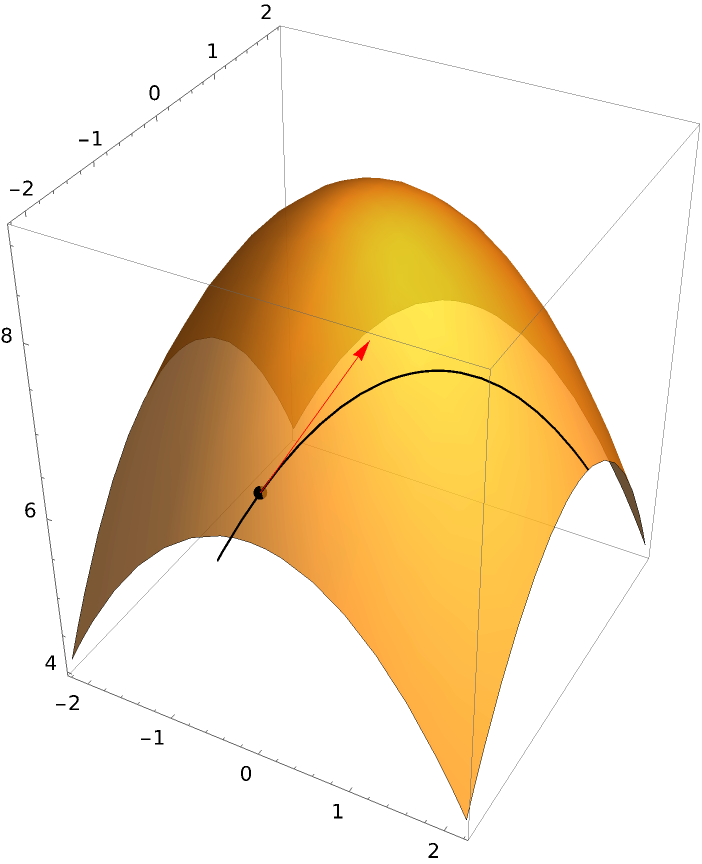### Scope (13)

Suppress drawing the section curve:

 In:=Out=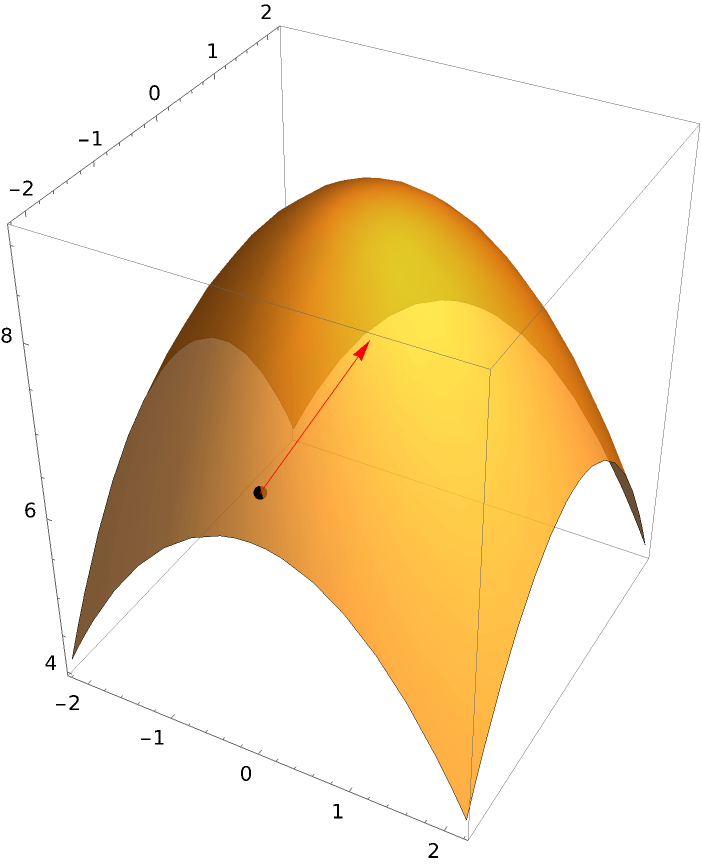Plot using two direction vectors:

 In:=Out=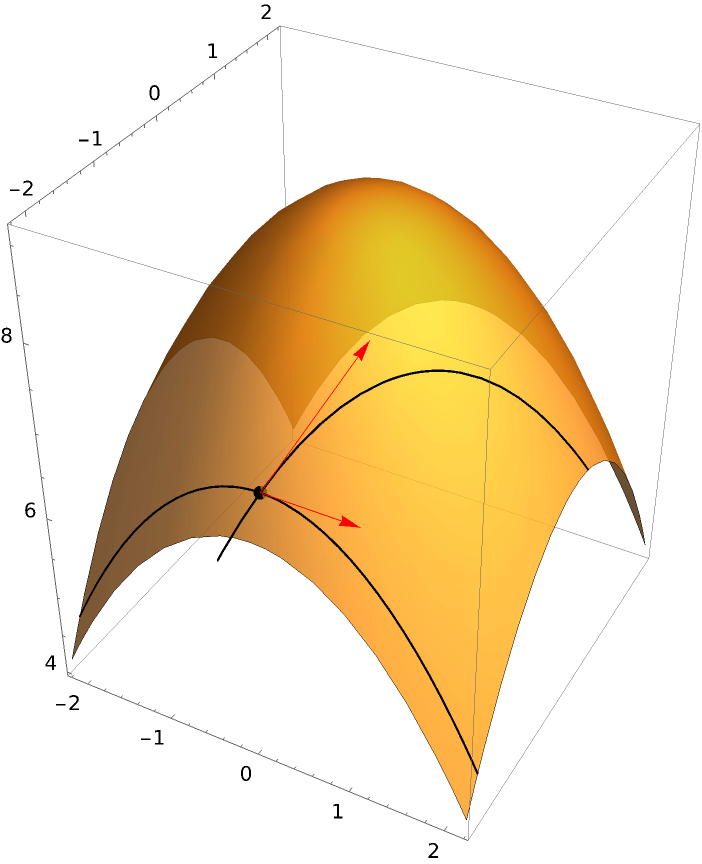Include a print of the values of the directional derivatives:

 In:=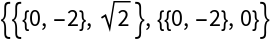Out=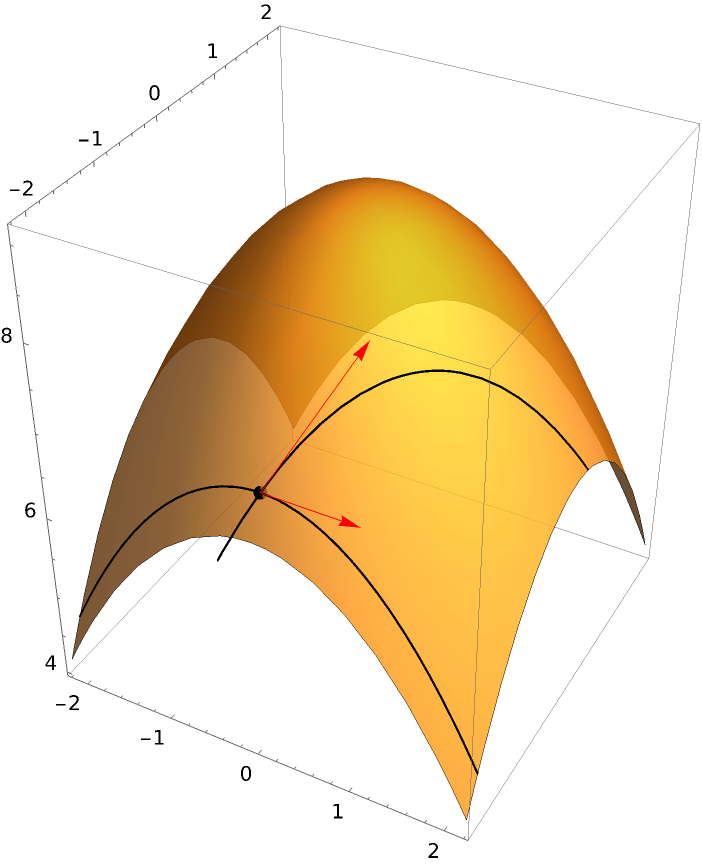Return just a print of the points and the directional derivatives:

 In:=Out=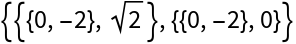Plot using two direction vectors and two points:

 In:=Out=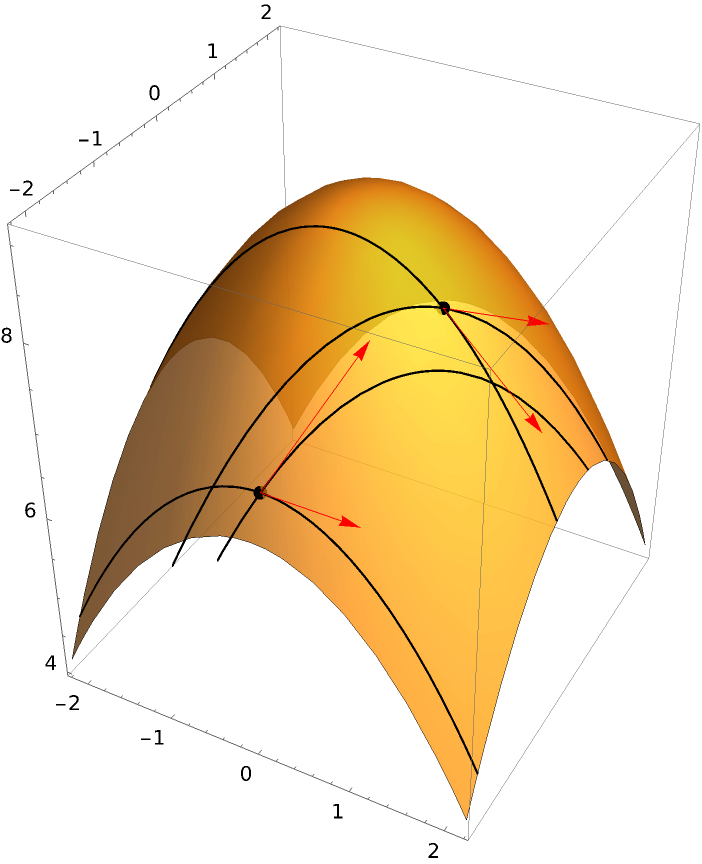If "DrawBaseVector" is set to True, then the vector determining the direction is plotted at the bottom of the plot:

 In:=Out=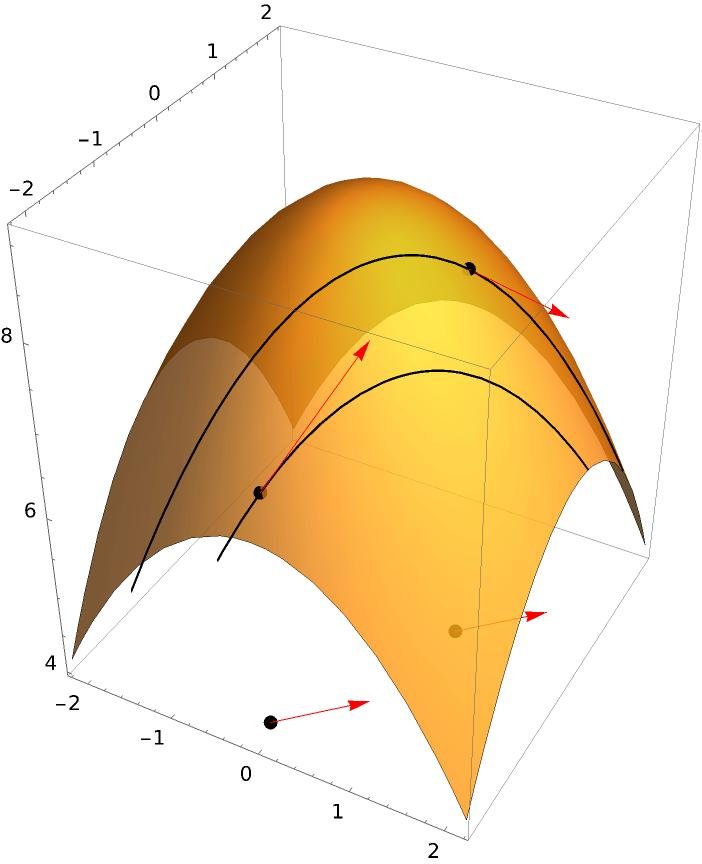Include the plane determining the section along with the base vector in the plot:

 In:=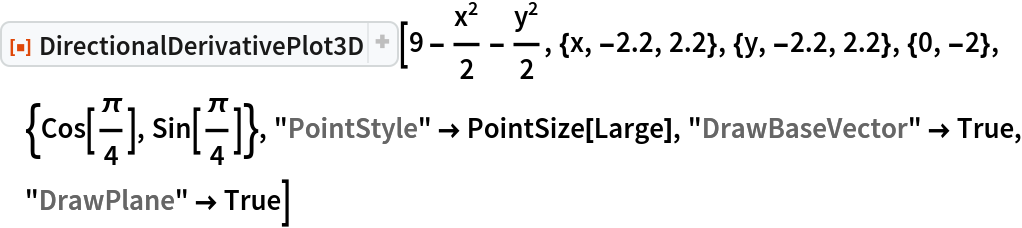Out=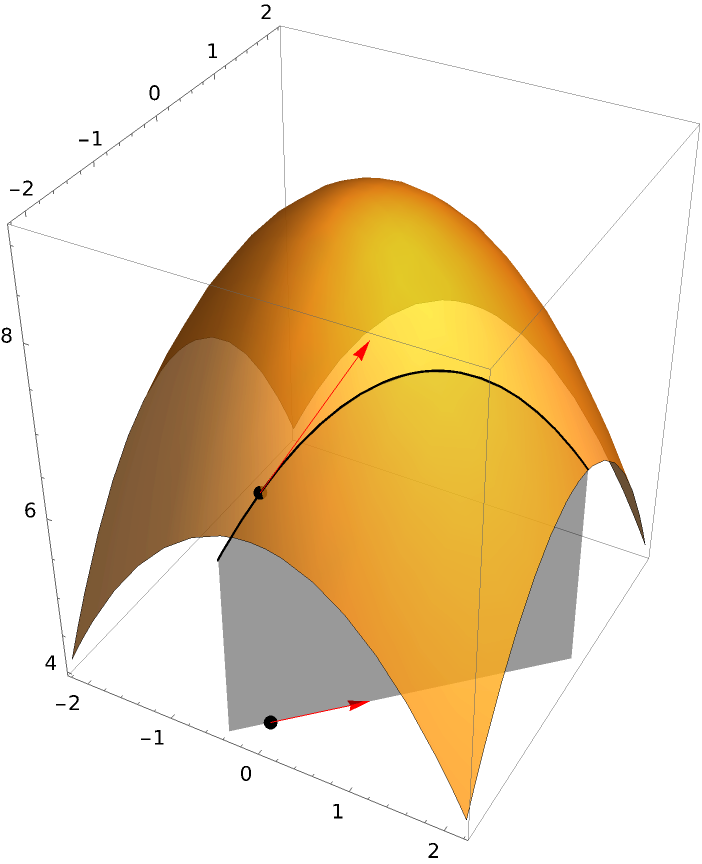The option "BaseVectorPosition" allows the vector to be plotted in any horizontal plane:

 In:=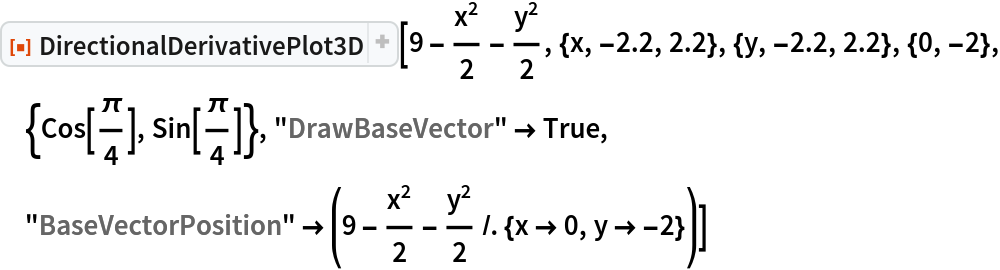Out=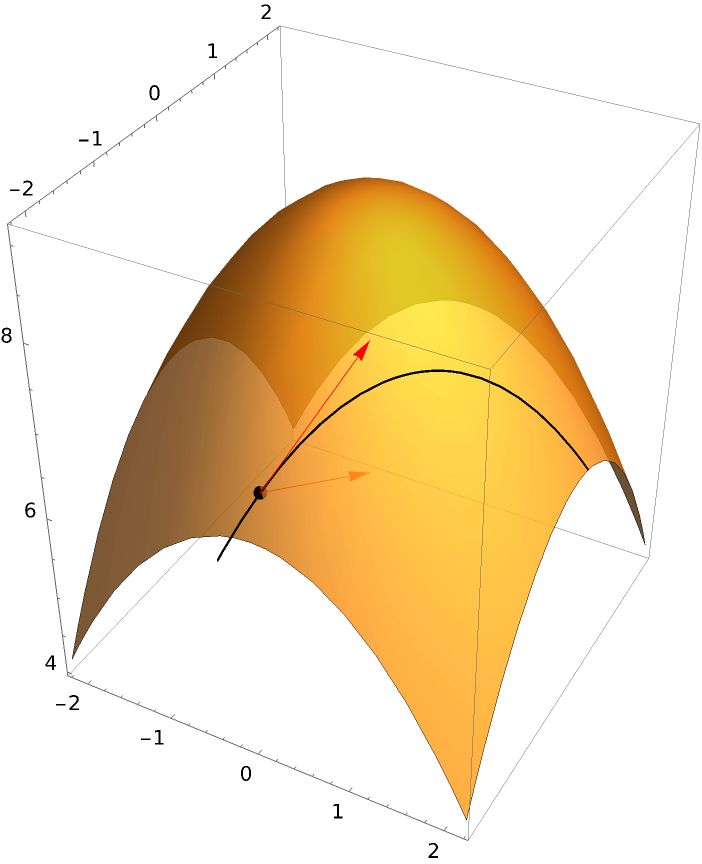Set a style for the vectors:

 In:=Out=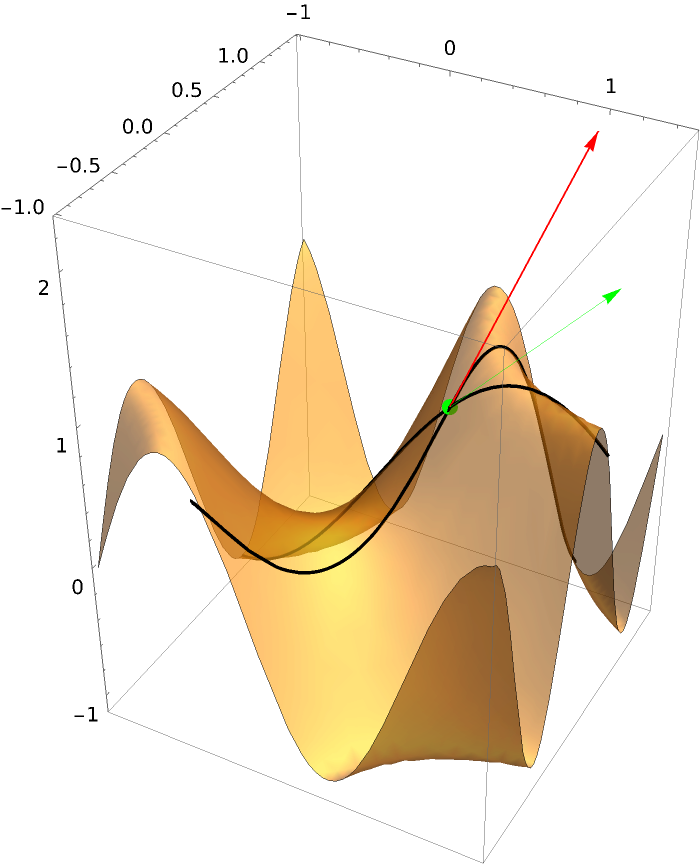Add the base vectors and lower their position:

 In:=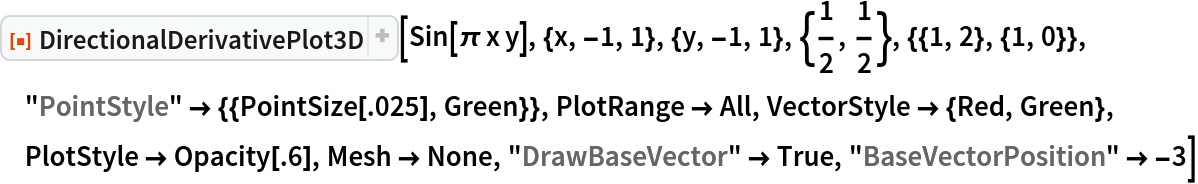Out=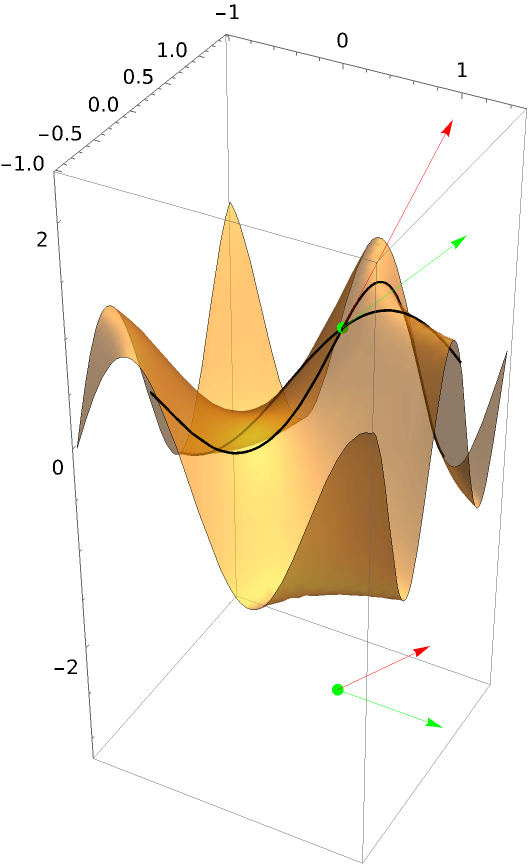A function requiring both "UseLimit” and ResourceFunction["UseRealRoots"]:

 In:=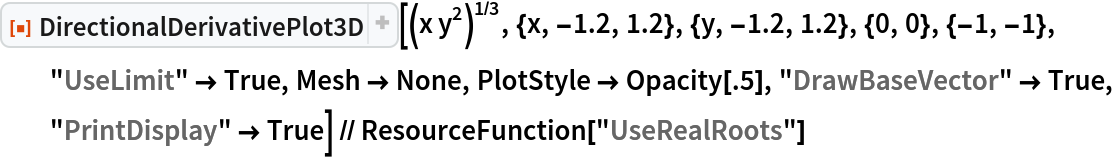Out=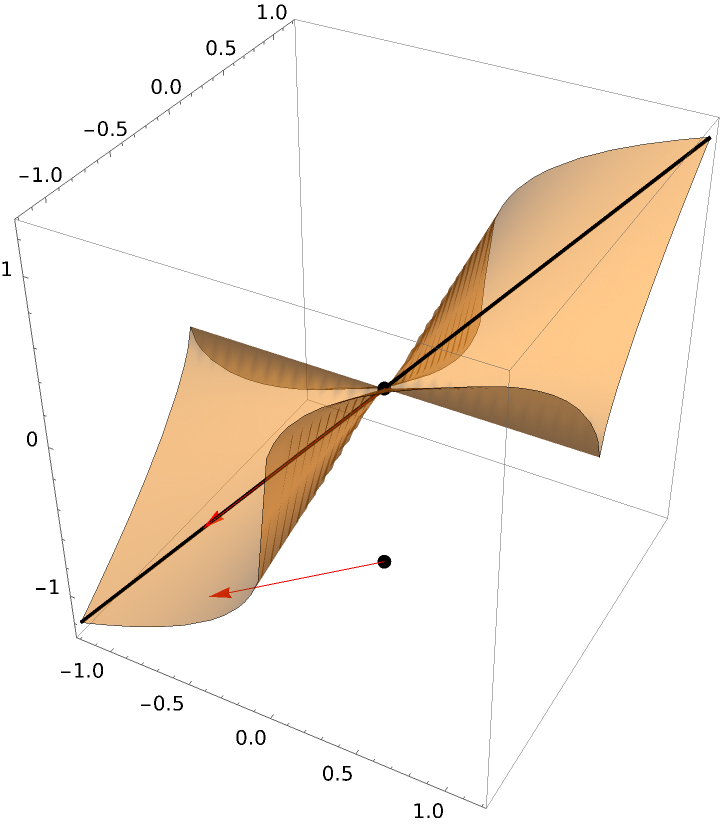Without using ResourceFunction["UseRealRoots"]:

 In:=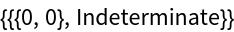Out=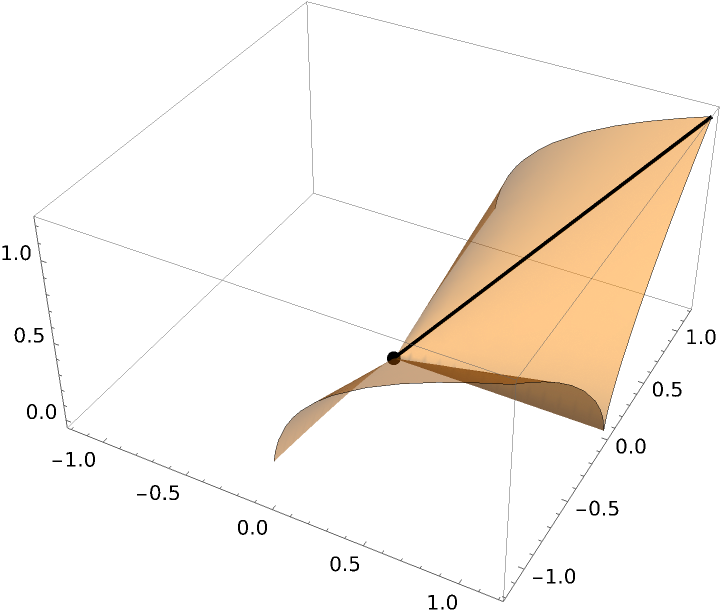Without using the option "UseLimit":

 In:=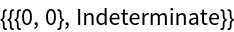Out=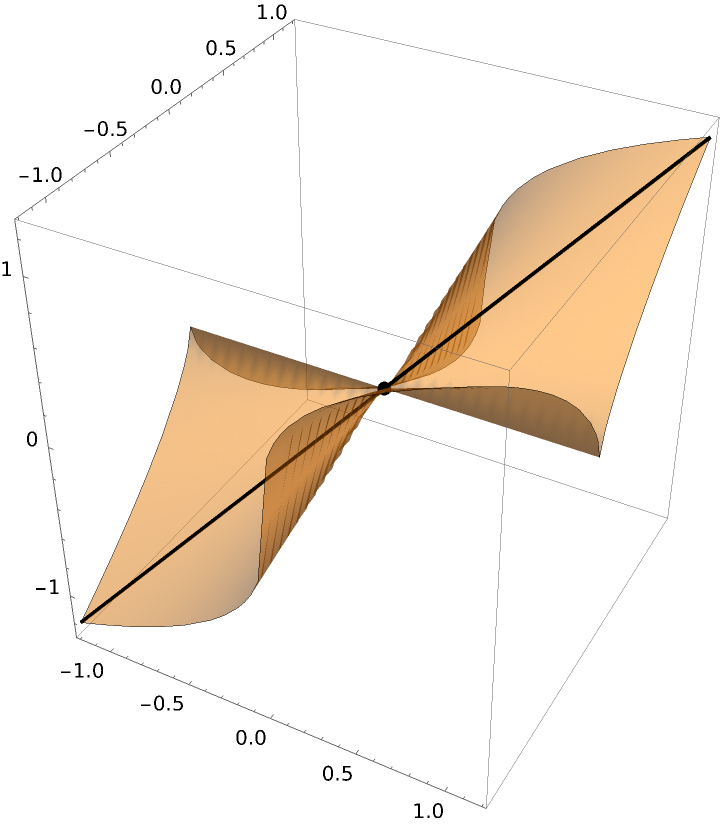Another function requiring the option "UseLimit” and ResourceFunction["UseRealRoots"]:

 In:=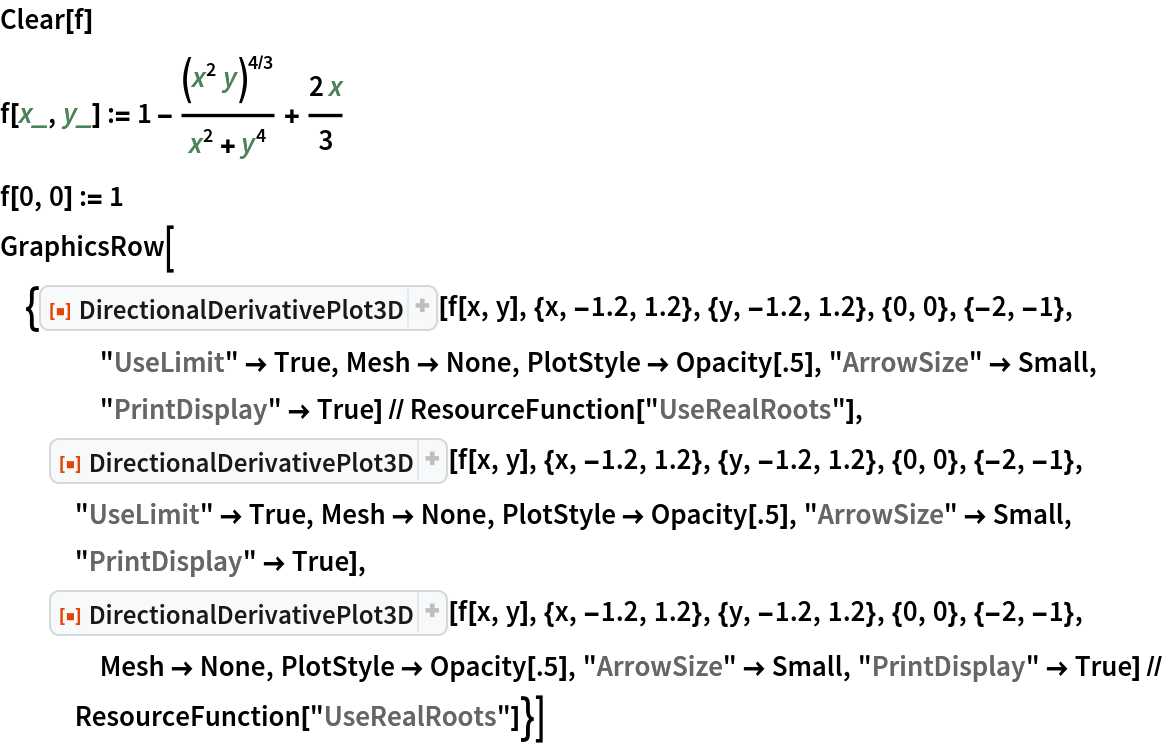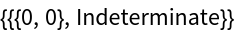Out=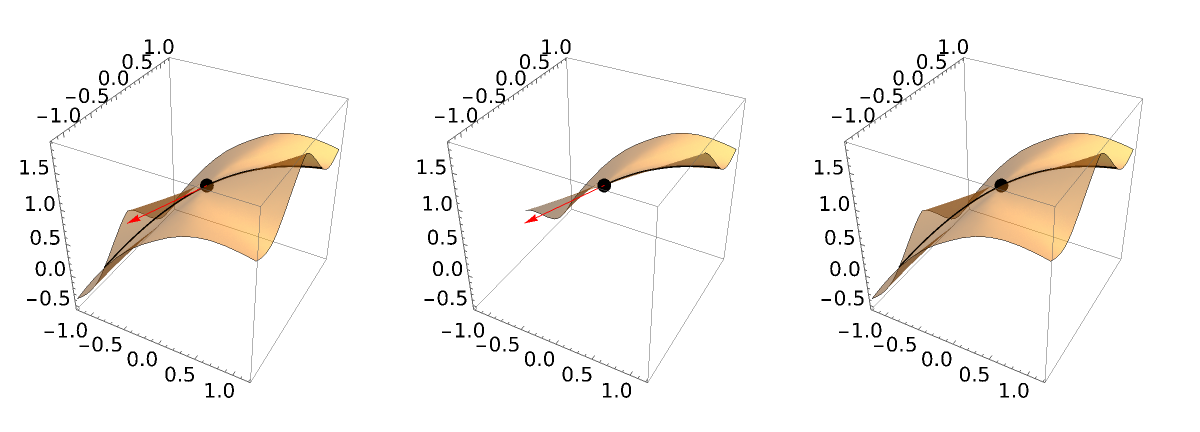## Publisher

Dennis M Schneider

## Version History

• 1.1.0 – 11 July 2022
• 1.0.1 – 15 January 2021
• 1.0.0 – 16 September 2020